Next: Worked example 9.3: Spinning Up: Angular momentum Previous: Worked example 9.1: Angular

## Worked example 9.2: Angular momentum of a sphere

Question: A uniform sphere of mass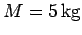and radius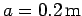spins about an axis passing through its centre with period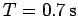. What is the angular momentum of the sphere?

Answer: The angular velocity of the sphere is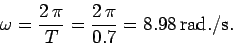The moment of inertia of the sphere is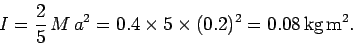Hence, the angular momentum of the sphere is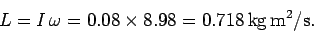Richard Fitzpatrick 2006-02-02